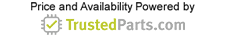Industrial Electronics

06 December 2022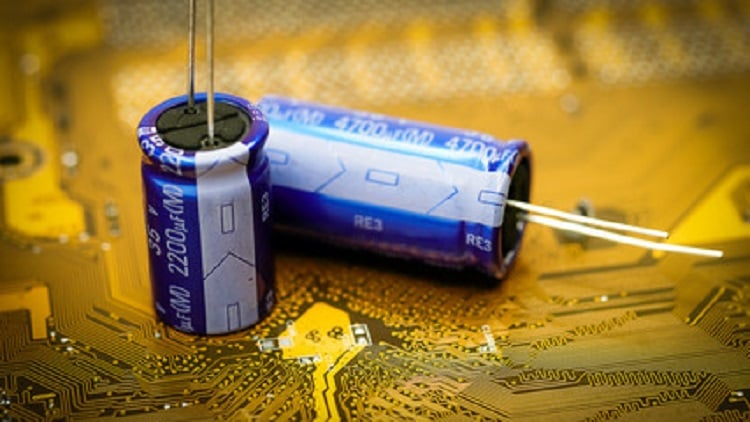Figure 1: Engineers and procurement professionals face several challenges when working with an electrical system featuring capacitors. Source: surasak/Adobe Stock

Capacitors are essential passive components that store charges and dissipate the stored energy to components on demand. They are used in a broad range of applications, including radars, pulsed lasers, rail guns and accelerators, among others.

However, while seemingly simple, engineers and procurement professionals face several challenges when working with an electrical system featuring capacitors. For instance, some procurement professionals do not understand the color-coding on capacitors.

This article presents answers to four frequently asked questions about capacitors. It will serve as a guide for those looking to understand capacitor color-coding and how to discharge capacitors.

## How does one read and understand capacitor color codes?

Every capacitor has colors or alphanumeric characters on its body, which provides information about its capacitance values, tolerances and voltage. To help better understand the capacitor code scheme, Figure 1 illustrates a Type L capacitor marked with five layers of colors.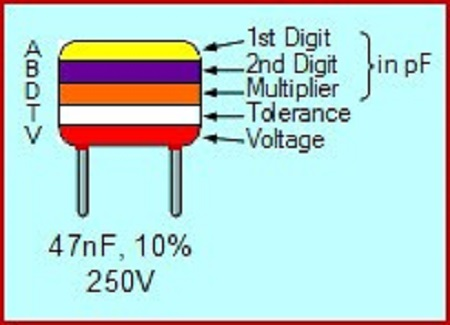Figure 1: Capacitor color code. Source: Elprocus

Each color on the capacitor color band represents a number (or digit), which can be read from Table 1 below. The first three digits allow readers to determine the capacitance value, while the fourth and fifth digits represent the tolerance and voltage values, respectively.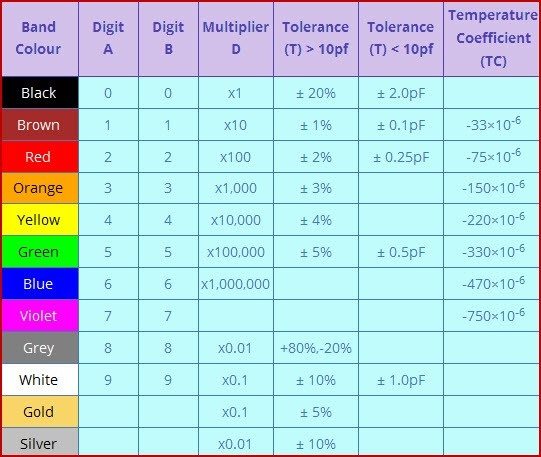Table 1: Color-coding scheme table for capacitors. Source: Elprocus

For instance, in the Type L capacitor illustration in Figure 1, the first color of the band is yellow, so the first digit is obtained to be 4. Likewise, the second color of the band is violet, so the second digit is obtained to be 7.

The third color of the band (which represents the multiplier) is orange, so the multiplier is obtained to be 1,000. Hence, the capacitance value can be obtained as shown below: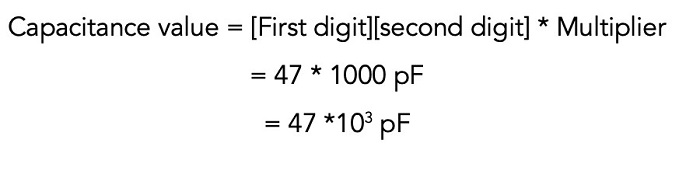Where:

Next, the fourth color of the band is white, so the tolerance is obtained to be ±10%. Finally, the fifth color of the band is red, so the voltage rating of the capacitor is obtained from Table 2 to be 250 V.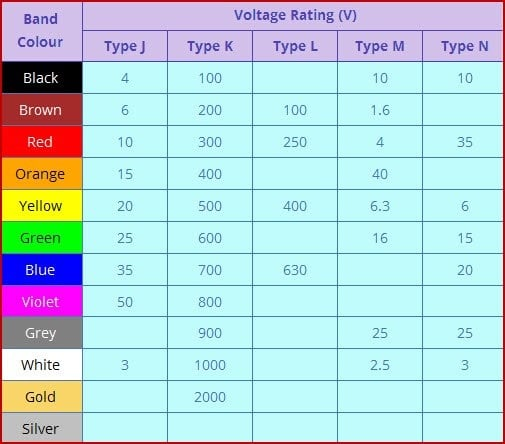Table 2: Voltage rating chart for capacitor color coding. Source: Elprocus

Compiling the above data, we have a capacitor with a capacitance of 47 × 103 pF ±10% and a voltage rating of 250 V.

## How does one measure capacitance using a multimeter?

Engineers can also determine the capacitance of a capacitor using a multimeter. A multimeter is an electrical instrument that is used to measure several electrical properties like voltage, current, resistance and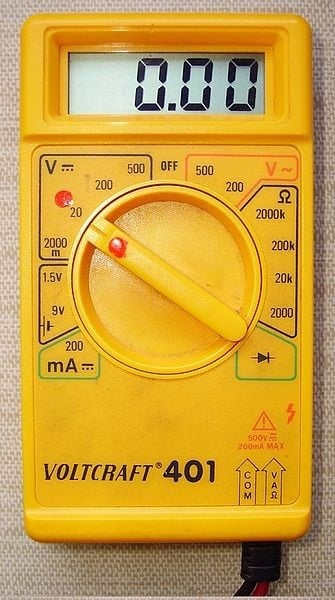Figure 2: A digital multimeter. Source: Cjp24/CC SA 3.0 capacitance. To measure capacitance using a multimeter, take note of the following steps:

1. Set the multimeter by plugging the black lead into the COM port and setting the dial to point to the capacitance symbol (–| (–).

2. Place the leads of the multimeter on the terminals of the capacitor and read the capacitance value displaced on the screen of the device.

Note: Avoid touching the terminals of the capacitor as it might interfere with the multimeter’s accuracy. In addition, it is important to discharge the capacitor or remove it from the circuit before measuring its capacitance.

## How does one discharge a capacitor?

Because capacitors can store (and hold) charges for a long time, they are usually still charged even after the electrical device containing the capacitors is powered off. As a result, technicians looking to perform maintenance on such electrical circuits might experience electrical shocks if they contact the capacitor's terminals.

Discharging a capacitor mitigates the risks of these electric shocks. The process involves releasing the charges stored in the capacitor, and it can be performed using a bleeder resistor. This resistor acts as a load by drawing (and dissipating) the stored energy in the capacitor.

However, when choosing a bleeder resistor, it is important to pay attention to the resistance and wattage of the resistor. For instance, resistors with small resistance allow more current to flow through them, discharging more quickly than units with higher resistance ratings. As a rule, a 2 kΩ, 5 W rated resistor is sufficient for most capacitor discharge applications. But for capacitors with higher voltage ratings (say greater than 400 V), engineers might want to consider using a 20 kΩ resistor.

## How long does the capacitor discharge process take?

Capacitor discharge time (or time constant) is the time after which the voltage of the capacitor should drop to one-third of its initial value. It can be calculated using:Where:

C = capacitance value of the capacitor

R = resistance of the bleeder resistor

Note: Measure the voltage reading across the lead of the capacitor after the discharge using a multimeter. If the reading is not close to 0 V, it implies the capacitor needs more time for discharge.

## Conclusion

While this article presents answers to four frequently asked questions about capacitors, there are several other challenges an electrician might face when working on a circuit containing capacitors. Therefore, engineers are advised to reach out to capacitor manufacturers to discuss their challenges and application needs.